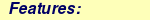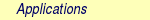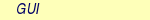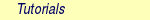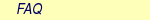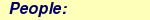MOLCAS manual:Next: 8.27 mckinley (a.k.a. denali) Up: 8. Programs Previous: 8.25 LoProp

Subsections

# 8.26 mbpt2

## 8.26.1 Description

The MBPT2 program of the MOLCAS program system computes the second order correlation energy and the reference weight for a closed-shell Hartree-Fock reference wave function, based on a Møller-Plesset partitioning of the Hamiltonian and canonical orbitals.

If SEWARD performed a Cholesky decomposition of the two-electron integrals prior to running the MBPT2 program, Cholesky vectors will be employed for computing the second order energy correction. This is done by first transforming the Cholesky vectors to MO basis and subsequently computing the (ai|bj) integrals. These integrals are either computed, stored on disk, and then read back in to memory during the energy evaluation (i.e. mimicking a conventional calculation) or they may be computed on-the-fly. The user may choose either algorithm through the Cholesky-specific options described below.

If SEWARD did not perform a Cholesky decomposition, the transformation of the two-electron integrals in AO basis (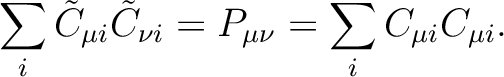, where N is the number of basis functions) to the exchange operator matricesin MO basis (matrices of size V2, where O and V denote the number of occupied and virtual orbitals, respectively), is either done conventionally, using the two-electron integral file ORDINT, which was generated in a previous step by the SEWARD integral code.

## 8.26.2 Dependencies

The MBPT2 program requires the communications file RUNFILE. It contains specifications processed by SEWARD, the Hartree-Fock canonical orbitals, eigenvalues and energy generated by SCF. For Cholesky-based calculations, all Cholesky related files (see the manual pages for SEWARD) must be available, whereas for conventional (not integral-direct) calculations the two-electron integral file ORDINT is required. Hence, before running MBPT2, a SEWARD and a SCF run have to be performed.

## 8.26.3 Files

### 8.26.3.1 Input files

MBPT2 will use the following input files: ONEINT, ORDINT,RUNFILE. For Cholesky runs: CHVEC, CHORST, CHRED and CHOR2F (for more information see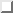).

### 8.26.3.2 Output files

 File Contents RUNFILE File for communication of auxiliary information.

## 8.26.4 Input

Below follows a description of the input to MBPT2. The input for each module is preceded by its name like:

&MBPT2

No compulsory keywords are required for MBPT2. The reference statement mentioned above is sufficient for a default MBPT2 run.

### 8.26.4.1 Optional keywords

 Keyword Meaning TITLe The line following this line is regarded as a title line PRINt Specifies the general print level of the calculation. An integer has to be supplied as argument. The default value, 0, is recommended for production calculations. FREEze Specifies the total number of frozen occupied orbitals. The lowest-energy occupied orbitals are then automatically identified and frozen. The keyword takes as argument one integer. Incompatible with the FROZen keyword. FROZen Specifies the number of frozen occupied orbitals in each of the irreducible representations (irreps) of the subgroup of D2h in which the system is represented. The counting of the orbitals follows the increasing orbital energy within each irrep, with those orbitals being frozen first that correspond to lowest orbital energies. The keyword takes as argument nIrrep (# of irreps) integers. Incompatible with the FREEze keyword. Default is to freeze non-valence orbitals. DELEted Specifies the number of deleted orbitals in each of the irreducible representations (irreps) of the subgroup of D2h in which the system is represented. The counting of the orbitals follows the decreasing orbital energy within each irrep, with those orbitals being deleted first that correspond to highest orbital energies. The keyword takes as argument nIrrep (# of irreps) integers. NOTE: Those orbitals, which have been deleted already in the SCF calculation (cf. SPDElete, OVLDelete of the SCF program description) are never seen by the MBPT2 program and hence are not to be deleted again with the present option. SFROzen Allows to specify specific orbitals to freeze in each of the irreducible representations (irreps) of the subgroup of D2h in which the system is represented. In the 1st line after the keyword the number of orbitals to freeze for each irrep is specified (nIrrep (# of irreps) integers). The nextnIrrep lines reference the orbitals to freeze for the related irrep, following an enumeration of the individual orbitals of 1, 2, 3,according to increasing orbital energy. Note that the orbital reference numbers obey the original ordering and also include those orbitals which may have been frozen already by the FROZen or FREEze options. If the corresponding irrep does not contain any specific orbitals to freeze (i.e. a zero was supplied for this irrep in the 1st line), no line orbital reference input line is supplied for that irrep. SDELeted Allows to specify specific orbitals to delete in each of the irreducible representations (irreps) of the subgroup of D2h in which the system is represented. In the 1st line after the keyword the number of orbitals to delete for each irrep is specified (nIrrep (# of irreps) integers). The nextnIrrep lines reference the orbitals to delete for the related irrep, following an enumeration of the individual orbitals of 1, 2, 3,according to increasing orbital energy. Note that the orbital reference numbers obey the original ordering. If the corresponding irrep does not contain any specific orbitals to freeze (i.e. a zero was supplied for this irrep in the 1st line), no line orbital reference input line is supplied for that irrep. GHOStdelete Excludes from PT2 treatment orbitals localized on ghost atoms. A threshold for this selection must be specified. LUMOrb Molecular orbital coefficients and energies read from INPORB file rather than RunFile. EREF Specifies the value of the reference energy. Available only in combination with LumOrb. Default value of the reference energy is set to zero. TEST If this keyword is specified the input is checked without performing any calculation. T1AM Singles amplitudes/energy introduced according to Thouless formula. An INPORB file containing MOs different from HF orbitals is required. LOVMp2 Freeze-and-Delete'' type of MP2, available only in connection with Cholesky or RI. An example of input for the keyword LOVM is the following: LovMP2 2  0.2  (nCenters,thrs) C1  N  (Center  labels) DoMP2 In this case, both occupied and virtual orbitals (localized by the program) are divided in two groups: those (A) mainly located on the two (symmetry independent) centers C1 and C2, and the remaining ones (B), which are obviously outside'' this region. The value of the threshold (between 0 and 1) is used to perform this selection (in the example, 20% of the gross Mulliken population of a given orbital on the specified atoms). By default, the MP2 calculation is performed only for the correlating orbitals associated with the region A (active site''). The keyword DoMP2 is optional and forces the program to perform also an independent MP2 calculation on the frozen region'' (B). Alternatively, one can specify the keyword VirAll in order to use all virtual orbitals as correlating space for the occupied orbitals of the active site. FNOMp2 Performs a Frozen Natural Orbital (FNO) MP2 calculation, available only in combination with Cholesky or RI integral representation. An example of input for the keyword FNOM is the following: FNOMp2 0.4 DoMP2 The keyword FNOM has one compulsory argument (real number in ]0,1]) specifying the fraction of virtual orbitals (in each irrep) to be retained in the FNO-MP2 calculation. The keyword DoMP2 is optional and used to compute the (estimated) correction for the truncation error. PRPT Multipole moments (dipoles and quadrupoles) are calculated and printed. The moments are calculated by using a variational one-particle MP2 density matrix. The calculation of the density matrix substantially increases the computational effort compared to an ordinary energy calculation. If the call to MBPT2 is followed by a LoProp call the variational MP2 density matrix will automatically be passed on to that module when this keyword is active. GRDT Variational one and two-particle MP2 densities are calculated to prepare for analytical gradient calculations. The default for subsequent gradient calculations are changed from numerical to analytical when this keyword is invoked. When using mbpt2 in a slapaf-loop with only C1 symmetry analytical gradients are automatically default and this keyword is not needed. grdt prints Multipole moments and prepare for LoProp in the exact same way as prpt. Use of this keyword therefore makes it redundant (but harmless) to also specify the keyword prpt. NOGRdt Disables the calculation of variational densities for analytical gradients. This is useful to cancel the implicit grdt added when using mbpt2 inside a slapaf-loop, if no analytic gradients are actually needed. Note that using the Numerical keyword in gateway already disables grdt, so nogrdt is only needed in some advanced situations.

### 8.26.4.2 Optional keywords specific to Cholesky calculations

Observe that these keywords are disregarded if the integrals were not Cholesky decomposed by SEWARD. Furthermore, they are disregarded for algorithm 0 (see below).
 Keyword Meaning CHOAlgorithm Takes as argument one positive integer specifying the algorithm to use for Cholesky MP2. Options: 0 [generate MO integrals on disk from Cholesky vectors], 1 [compute integrals on-the-fly, minimal operation count, level 2 BLAS], 2 [compute integrals on-the-fly, not minimal operation count, level 3 BLAS], Default is 2. VERBose Increases printing from the Cholesky MP2 routines, although not by much. Default is (almost) no printing. DECOmpose Requests Cholesky decomposition of the (ai|bj) integrals. Unless user-defined (see below), the threshold used is identical to that used by SEWARD for decomposing the two-electron integrals. Default is to not decompose. THRCholesky Specifies the threshold for (ai|bj) Cholesky decomposition. Takes as argument one real number. Default is the threshold used by SEWARD for decomposing the two-electron integrals. NODEcompose Turns off Cholesky decomposition of the (ai|bj) integrals. Default is to not decompose. SPAN Specifies the span factor used for (ai|bj) Cholesky decomposition. Takes as argument one real number. Default is the span factor used by SEWARD for decomposing the two-electron integrals. MXQUal Specifies the max. number of qualified diagonals treated during (ai|bj) Cholesky decomposition. Takes as argument one integer. Default is 10% of the max. rank of (ai|bj), although never more than 200. PRESort Presort the MO Cholesky vectors according to the batches over occupied orbitals. This will reduce the amount of I/O performed during on-the-fly assembly of the (ai|bj) integrals. This keyword is obsolete.

### 8.26.4.3 Limitations

The maximum number of selectively frozen SFRO or selectively deleted orbitals SDEL in each symmetry is limited to 50.
The limitations on the number of basis functions are the same as specified for SEWARD.

### 8.26.4.4 Input example

&MBPT2
Title
H2O:  O(9.5/4.2),  H(4/2)
*  The  lowest  energy  occupied  orbital  in  the  repr.  no.1  will  be  frozen  in
*  MBPT2  calculations.  The  number  of  representations  is  4  and  all  zeros
*  must  be  explicitly  given
Frozen
1  0  0  0
*  Two  highest  energy  external  orbitals  in  the  repr.  no.3  will  be  deleted
*  in  MBPT2  calculations.  The  number  of  representations  is  4  and  all
*  zeros  must  be  explicitly  given
Deleted
0  0  2  0
*  One  occupied  orbital  in  symmetry  no.1  will  be  additionally  frozen  by
*  using  the  SFRO  option.  Let  it  be  the  third  SCF  occupied  orbital  in
*  this  symmetry
sFrozen
1  0  0  0  //  Gives  the  number  of  frozen  orbitals  in  each  symmetry
3  //  Gives  the  frozen  orbital  reference  number  in  symmetry  no.  1
*  Two  external  orbitals  in  symmetry  no.1  and  one  external  orbital  in
*  symmetry  3  will  be  deleted.  In  symmetry  1  let  it  be  the  second  and
*  third  external  orbitals,  and  in  symmetry  3  the  third  (already  deleted
*  in  by  using  the  option  DELE)  external  orbital
sDeleted
2  0  1  0  //  Gives  the  number  of  orbitals  to  be  deleted  in  each  symmetry
2  3  //  Gives  the  reference  numbers  of  external  orbitals  in  sym.  1
3  //  Gives  the  reference  number  of  the  external  orb.  in  sym.  3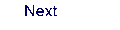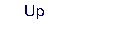Next: 8.27 mckinley (a.k.a. denali) Up: 8. Programs Previous: 8.25 LoProp